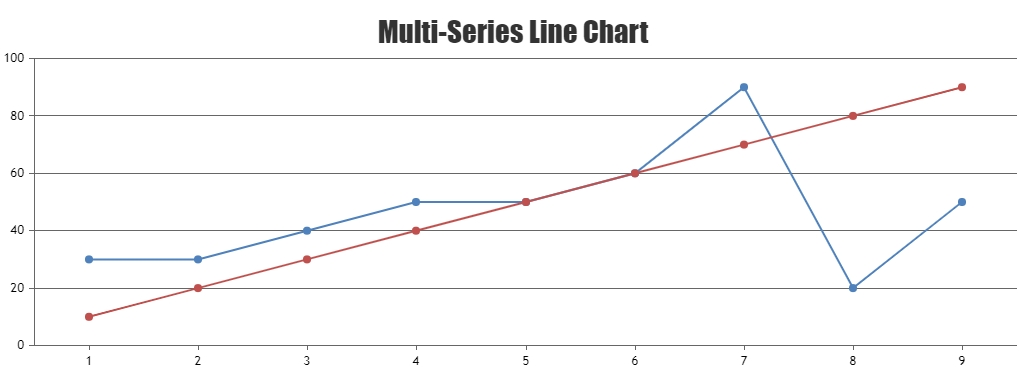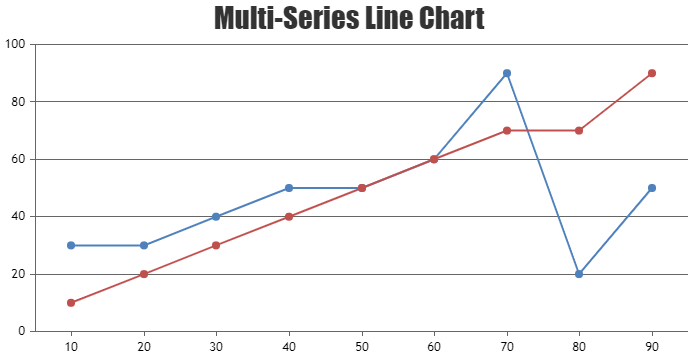Home Forums Chart Support Interpolate the missing data as last value in Multiseries chart

# Interpolate the missing data as last value in Multiseries chart

Viewing 4 posts - 1 through 4 (of 4 total)
• #36699

I have a problem with the Multi-Series line chart. I want to keep the missing value the same as the previous one.

https://jsfiddle.net/b5wmLoyp/2/

That means I don’t want the blue line to go straight from `1` to `3` like in the fiddle, I want it to go like below.I store the data only when they are changed in my system. So, `2` would look like same as `1` since there’s no data point that means it didn’t change.

How should I implement it?

#36719

You can loop through datapoints and add the missing datapoint before rendering the chart. Please find the code-snippet below.

``````for(i=0; i<chart.options.data.length; i++) {
var dps = chart.options.data[i].dataPoints;
for(var j = 1; j < dps.length; j++) {
if(dps[j].x > (j+1))
dps.splice(j, 0, {x: j+1, y: dps[j-1].y});
}
}``````

Also, check out this JSFiddle for complete working sample.—-
Manoj Mohan
Team CanvasJS

#36759

This does not meet with the request however on a picture it does.

Assume it’s a time series and the data increases with not a certain frequency, not like the above example is simple increases only one by one. But I want to compare the red data points with the blue one and if there’s no value in the other red one, go with a straight line instead of going linear…

That means comparing them with each other.

It is also hard to pre-assume how many different series I will have as it depends on the user’s query.

#36782

In order to add missing dataPoint on each series, you can loop through each dataSeries and find the missing dataPoints and push into respective dataSeries as shown in the code snippet below.

``````function addMissingDataPoints(chart) {
var missingValues = [];
for(var i = 0; i < chart.options.data.length; i++) {
missingValues[i] = [];
var dataSeriesToBeChecked = chart.options.data[i];
for(var j = 0; j < chart.options.data.length; j++) {
if(j == i) continue;
var currentDp = chart.options.data[j].dataPoints;
for(var k = 0; k < currentDp.length; k++) {
var dp = getPreviousDp(currentDp[k].x, dataSeriesToBeChecked.dataPoints);
if(dp === true)
continue;
missingValues[i].push(dp);
}
}
}

for(var i = 0; i < chart.options.data.length; i++) {
for(var j = 0; j < missingValues[i].length; j++) {
// push the missing values
chart.options.data[i].dataPoints.push(missingValues[i][j]);
}
//sorting the dataPoints so that missing values get adjusted to appropriate place
chart.options.data[i].dataPoints.sort((a,b) => (a.x < b.x ? -1 : (a.x > b.x ? 1 : 0)))
}
}

function getPreviousDp(xValue, dps) {
for(var i=0; i<dps.length; i++) {
if(dps[i].x == xValue) {
return true;
}
if(dps[i].x > xValue)
break;
}
return {x: xValue,  y: (i <= 0 ? null : dps[i-1].y)};
}``````

Please take a look at this JSFiddle for complete code.—-
Manoj Mohan
Team CanvasJS

Viewing 4 posts - 1 through 4 (of 4 total)

You must be logged in to reply to this topic.